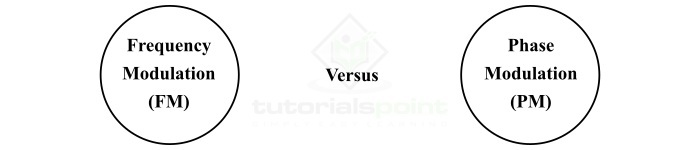# Difference between Frequency and Phase Modulations

In communication systems, modulation is a process of changing the characteristics of a data carrier wave so that it can cover the desired distance for data transmission. Modulation is a necessary process in communication systems to increase the reliability of data communication.

In the modulation process, an additional signal, called carrier signal, is generated that can go up to long distances, then the message signal is superimposed with this carrier signal, so that the carrier signal transmit the message signal over a long distance without getting affected by the external disturbances. This process is known as modulation.There are several types of modulation techniques such as amplitude modulation (AM), phase modulation (PM), frequency modulation (FM), etc. In this article, we will discuss the frequency modulation (FM) and phase modulation (PM), and the important differences between them. Let us start with a basic introduction of FM and PM.

## What is Frequency Modulation (FM)?

When the frequency of the carrier signal is varied in accordance with the instantaneous amplitude of the modulating signal while keeping amplitude and phase constant, it is called frequency modulation (FM). Frequency modulation is a type of angle modulation. Therefore, in the case of frequency modulation or FM, the frequency of the carrier signal is modified for data transmission. Frequency modulation can be divided into the following subtypes −

• Narrowband Frequency Modulation − This type of FM has a small bandwidth and modulation index. The narrowband frequency modulation is widely used in mobile communication systems.

• Wideband Frequency Modulation − This type of FM has infinite bandwidth and modulation index greater than 1. Wideband frequency modulation is extensively used in radio and TV communications.

The most important thing to be noted about the frequency modulation (FM) is that in this modulation technique, the amplitude and phase of the carrier wave remain constant.

## What is Phase Modulation (PM)?

Phase modulation (PM) is a type of the angle modulation technique. Phase modulation can be defined as the modulation process in which the phase of the carrier signal is changed in accordance with the instantaneous amplitude of the modulating signal. Thus, in the phase modulation technique, the phase of the carrier signal is modified for data transmission.

In the case of phase modulation, the amplitude and frequency of the carrier signal remains constant while only the phase of the carrier signal is changed. In the case of phase modulation, the modulation index is always more than 1. Phase modulation is widely used in several digital data transmission systems such as in WIFI, GSM, Satellite Communication, mobile communication, etc.

After discussing the basics of frequency modulation and phase modulation, let us now discuss the important differences between them.

## Difference between Frequency Modulation and Phase Modulation

The following table gives the important differences between frequency modulation (FM) and phase modulation (PM) −

Parameter

Frequency Modulation

Phase Modulation

Abbreviation

Frequency modulation is abbreviated as FM.

Phase modulation is abbreviated as PM.

Definition

Frequency modulation is a modulation technique in which frequency of the carrier signal is modified according to the modulating signal to send data and information.

Phase modulation is a type of modulation in which phase of the carrier signal is modified according to the modulating signal to send data and information.

Constant parameter of carrier signal

In frequency modulation, the amplitude and phase of the carrier wave remain constant.

In phase modulation, the amplitude and frequency of the carrier wave remain constant.

Variable parameter of carrier signal

In frequency modulation, the frequency of the carrier signal is variable.

In phase modulation, the phase of the carrier signal is variable.

Types

Frequency modulation is of two types namely, Narrowband Frequency Modulation and Wideband Frequency Modulation.

The important types of phase modulation include Phase Shift Keying (PSK), Binary Phase Shift Keying (BPSK), Quadrature Phase Shift Keying (QPSK), etc.

Signal to noise ratio

The signal to noise ratio of frequency modulation is better than phase modulation.

The signal to noise ratio of phase modulation is poor than that of the frequency modulation.

Noise immunity

Frequency modulation has better noise immunity.

Phase modulation is relatively less immune than frequency modulation.

Frequency deviation

In frequency modulation, the frequency deviation is directly proportional to the modulating voltage only.

In phase modulation, the frequency deviation is directly proportional to both modulating frequency and modulating voltage.

In frequency modulation, the quality of the received signal is high.

In phase modulation, the quality of the received signal is poor.

Application

Phase modulation is widely used in mobile systems such as the walky talkies in police vans, ambulance, taxicabs, etc.

## Conclusion

In conclusion, in frequency modulation, the frequency of the carrier wave is modified to transmit the data and information over communication channel, whereas in phase modulation, the phase of the carrier signal is changed for data transmission. However, both frequency modulation and phase modulation are the types of angle modulation. Hence, it is possible to receive FM signal on a PM receiver and vice-versa.

Updated on: 27-Mar-2023

3K+ Views